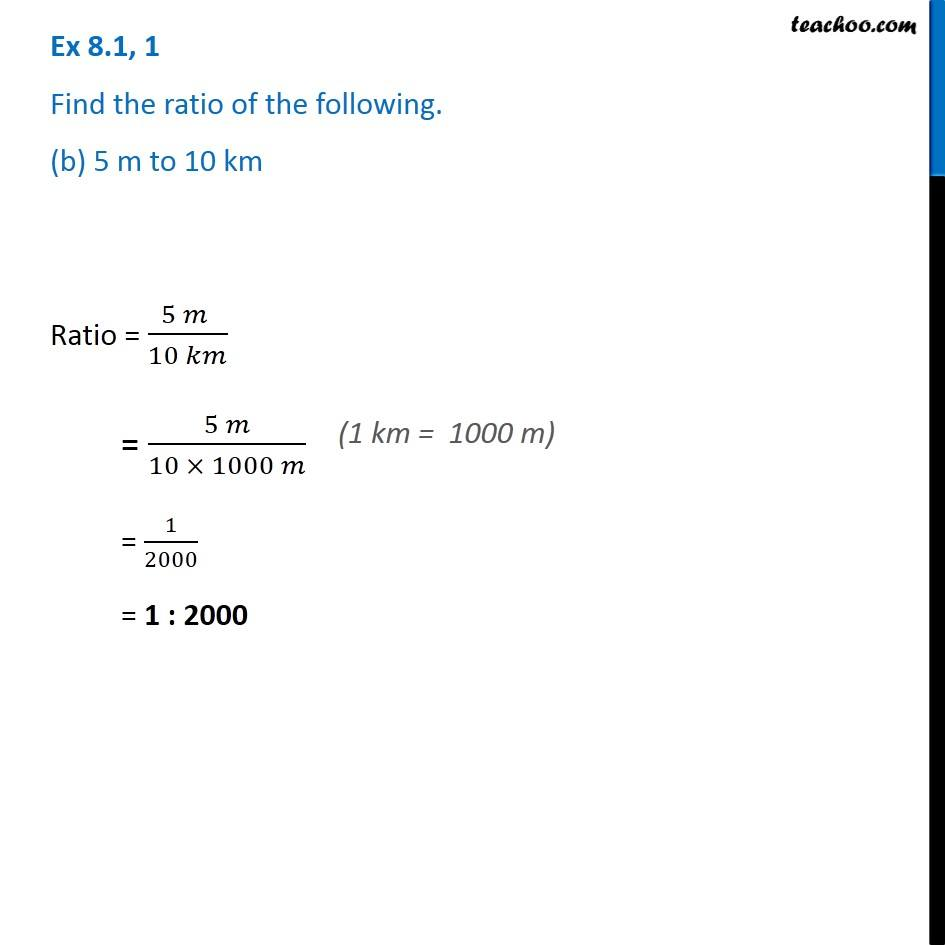Ex 8.1

Chapter 8 Class 8 Comparing Quantities
Serial order wiseGet live Maths 1-on-1 Classs - Class 6 to 12

### Transcript

Ex 8.1, 1 Find the ratio of the following. (b) 5 m to 10 kmRatio = (5 𝑚 )/(10 𝑘𝑚) = (5 𝑚)/(10 × 1000 𝑚) = 1/2000 = 1 : 2000 (1 km = 1000 m)